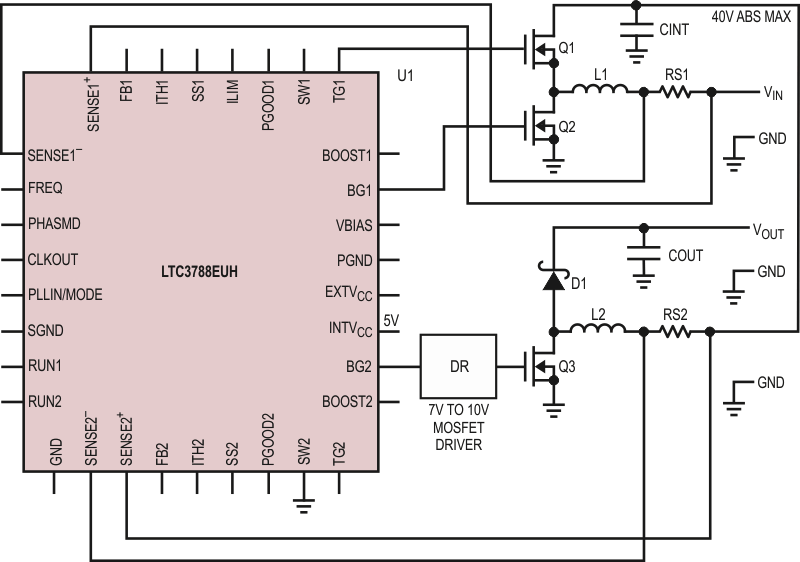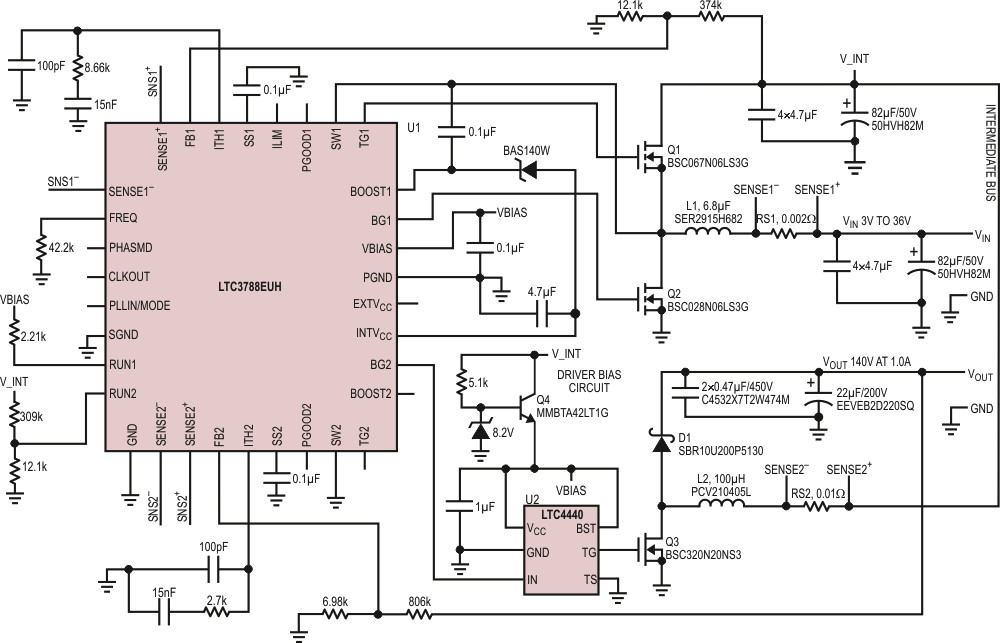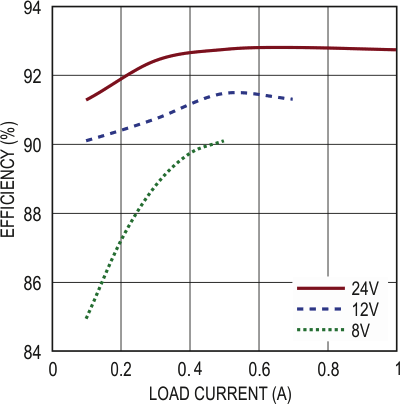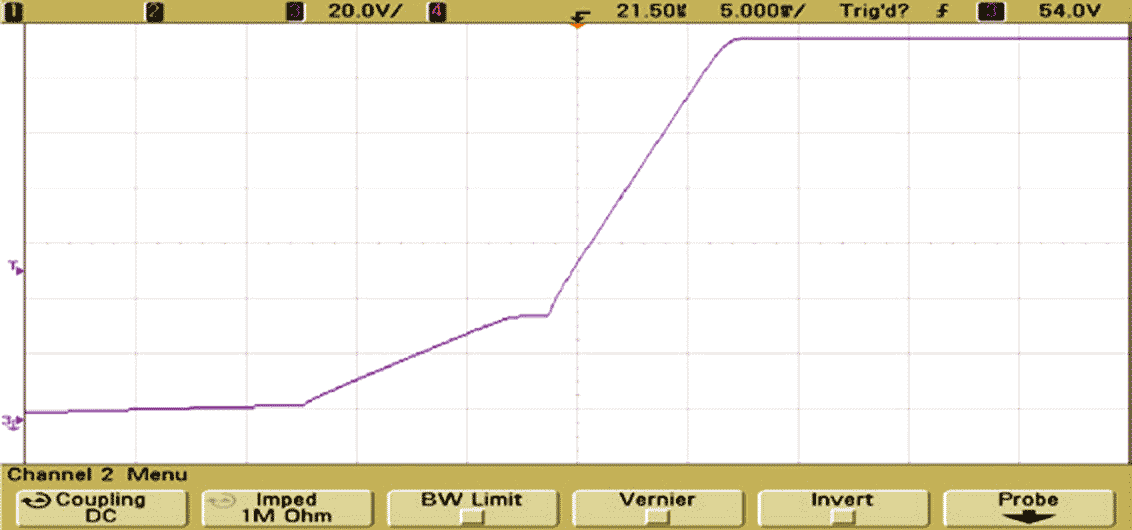# Easily Boost 12 V to 140 V with a Single Converter IC

## Linear Technology LTC3788

Victor Khasiev, Linear Technology

Design Note 1033

## Introduction

Generating a high voltage from a much lower voltage presents a number of challenges for the classical single stage boost topology. For instance, the maximum duty cycle limitation of a boost controller may not allow the required step-up ratio. Even if it does, there is often a sharp decrease in efficiency at high duty cycles. The duty cycle can be shortened by choosing discontinuous mode of operation, but this leads to high peak input current, higher losses and EMI challenges.

An alternative to a single boost converter is a 2-stage boost converter, where the first stage produces an intermediate voltage and the second stage boosts to the final high voltage. A 2-stage converter can be produced with a single controller IC, such as the LTC3788, a high performance 2-phase dual output synchronous boost controller, which drives all N-channel power MOSFETs.

The LTC3788 can be configured such that the first boost stage takes advantage of its synchronous rectification feature, which maximizes efficiency, reduces power losses and eases thermal requirements. The maximum output voltage of this controller is 60 V, when using synchronous rectification. If greater than 60 V is required, the second stage can be designed to run non-synchronously, as described below.

## 2-Stage Boost Produces 140 V from 12 V

The block diagram in Figure 1 shows the LTC3788 in a 2-stage boost configuration. This block diagram also reveals a few caveats that must be observed in this design:

• The output of the first stage (Q1, CINT) is connected to the input of second stage (RS2, L2). The output of the first stage should not exceed 40 V, because maximum absolute rating of the SENSE pins is 40 V.

• The gate drive voltage of 5 V is suitable for logic level MOSFETs, but not for high voltage standard MOSFETs, with typical gate voltages of 7 V to 12 V. The external gate driver DR, controlled by the BG2 signal can be used as shown here to drive high voltage standard MOSFETs.

• To generate an output voltage above maximum limit of 60 V, the synchronous rectification MOSFET is replaced by a single diode D1.Figure 1. Block Diagram of LTC3788-Based 2-Stage Boost Converter.

Figure 2 shows the complete solution. Transistors Q1, Q2, and inductor L1 compose the first stage, which generates an intermediate bus voltage of 38 V. The first stage employs synchronous rectification for maximum efficiency. The output of the first stage is connected as input to the second stage, comprised of Q3, D1, L2. The output of second stage produces 140 V at 1 A.Figure 2. Full Schematic of 2-Stage 140 V Output, 1 A Boost Converter.

Transistor Q3 is standard level MOSFET, driven by the LTC4440. Here, an LDO, based on transistor Q4, biases the gate driver, but a switching regulator can be employed instead (such as one built around the LTC3536) to further increase overall efficiency.Figure 3. Efficiency of the 2-Stage Converter in Figure 2 [VIN 8 V to 24 V, VOUT 140 V].

This solution features an input voltage range from 3 V to 36 V, nominal 12 V. To decrease components’ thermal stress, the output current should be reduced when the input voltages falls below 10 V. Figure 3 shows measured efficiency, and Figure 4 shows the start-up waveforms. A 93% efficiency is shown with VIN = 24 V and with the 140 V output loaded from 0.4 A to 1 A. This converter can operate at full load with no airflow.Figure 4. Start-Up Waveforms [from VIN 12 V to VOUT 140 V at 1 A].

## Conclusion

LTC3788 is a high performance 2-phase dual output synchronous boost controller, suitable for high power, high voltage applications. Its dual outputs can be used in tandem to achieve extremely high step-up ratios to high voltages.

cds.linear.com